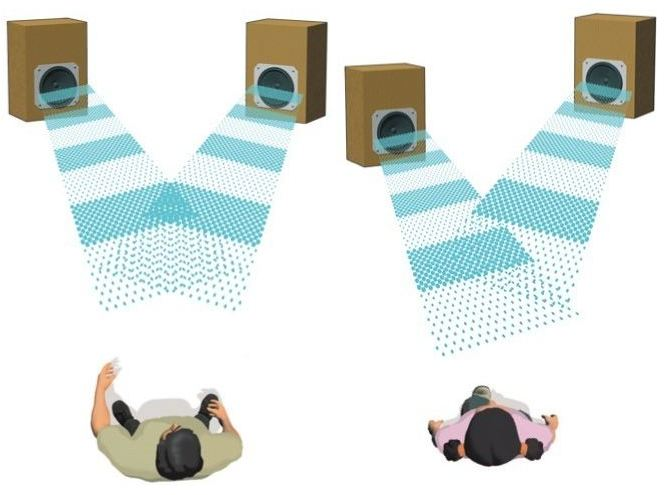# Wave InterferenceTwo speakers are generating sounds with the same phase, amplitude, and wavelength. The two sound waves can make constructive interference, as above left. Or they can make destructive interference, as above right. If we want to find out the exact position where the two sounds make destructive interference, which of the following do we need to know?

a) the wavelength of the sound waves
b) the distances from the two speakers
c) the speed of sound generated by the two speakers

×